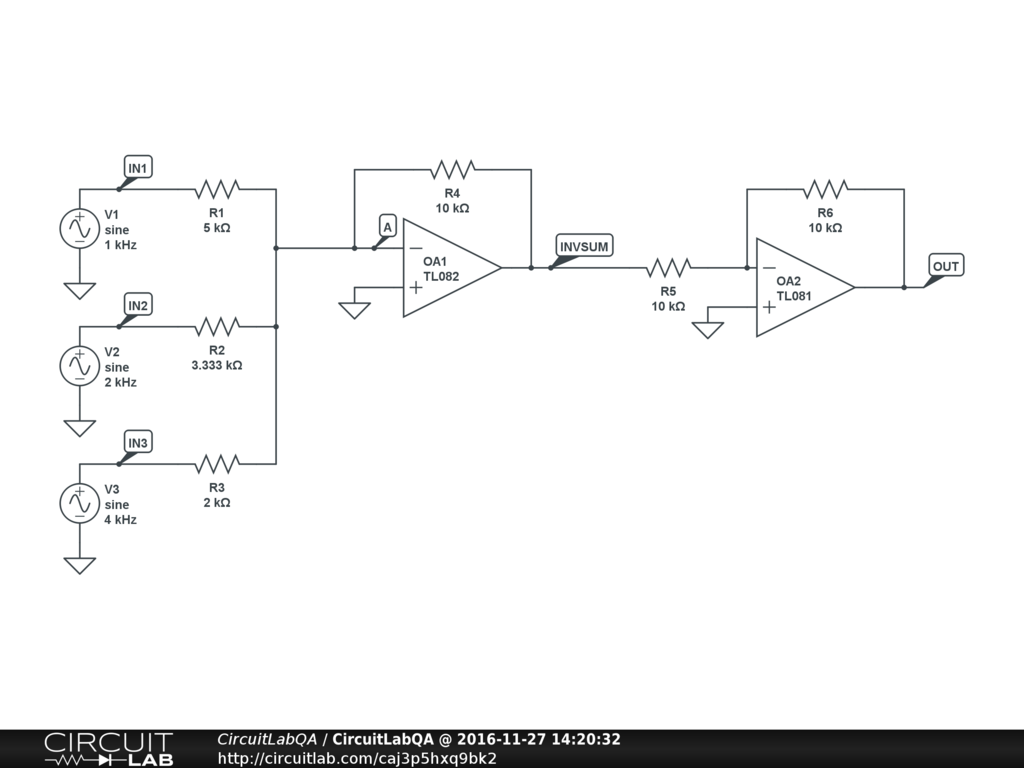## Summing three inputs with op amp SOLVED

 I have to design an amplifier that has $$V_{out} = 2 V_1 + 3 V_2 + 5 V_3$$ supposed to use two op-amps and as many resistors as needed. But i cant figure out resistors by vivgre78 November 25, 2016

 How about this:There are two stages: the first stage (OA1 and R1,R2,R3,R4) does the weighted summing. The second stage (OA2 and R5,R6) is simply an inverting gain of -1. The resistors R1,R2,R3 are chosen so as to set the individual gains for each input. Because node A is a virtual ground, each input voltage source V1,V2,V3 contributes a current proportional to its voltage: $i_1 = \frac {V_1} {R_1}$ and so on. These currents are added together, $i_{total} = i_1 + i_2 + i_3$ and flow through resistor R4. The resulting voltage $$V_{INVSUM} = -R_4\big(\frac {V_1} {R_1} + \frac {V_2} {R_2} + \frac {V_3} {R_3}\big)$$ So arbitrarily picking $R_4 = 10k\Omega$ then we have $2=\frac {10k\Omega} {R_1}$ or $R_1 = \frac {10k\Omega} {2}$ -- and so on for the other two inputs, $R_2 = \frac {10k\Omega} {3}$, $R_3 = \frac {10k\Omega} {5}$. In practice you could adjust all of these to find real resistor values that you could actually buy or adjust with a potentiometer. The second stage just inverts $V_{INVSUM}$, so $$V_{OUT} = R_4\big(\frac {V_1} {R_1} + \frac {V_2} {R_2} + \frac {V_3} {R_3}\big)$$ Click the circuit & try the simulation! ACCEPTED +1 vote by mrobbins November 27, 2016

CircuitLab's Q&A site is a FREE questions and answers forum for electronics and electrical engineering students, hobbyists, and professionals.

We encourage you to use our built-in schematic & simulation software to add more detail to your questions and answers.

Acceptable Questions:

• Concept or theory questions
• Practical engineering questions
• “Homework” questions
• Software/hardware intersection
• Best practices
• Design choices & component selection
• Troubleshooting

Unacceptable Questions:

• Non-English language content
• Non-question discussion
• Non-electronics questions
• Vendor-specific topics
• Pure software questions
• CircuitLab software support

Please respect that there are both seasoned experts and total newbies here: please be nice, be constructive, and be specific!